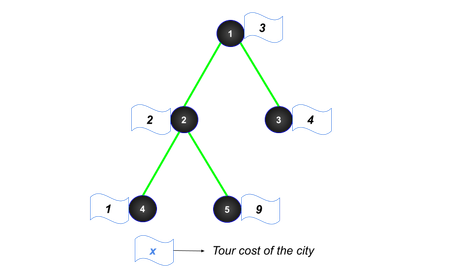# Budget TourashikurrahmanIntra AUST Programming Co...
Limits 3s, 512 MB

Dipu Bhai lives in a country called Chocoland. There are $n$ cities in Chocoland. The cities are numbered from $1$ to $n$. The cities of Chocoland can be represented as a tree.

There is a cost associated with every city. It is called the tour cost. Tour cost of $i^{th}$ city is denoted by an integer $a_i$, means Dipu Bhai has to pay exactly $a_i$ amount of money to visit the city $i$. Dipu Bhai has $m$ amount of money. He wants to visit the cities of Chocoland. But he has a limited money and can not visit all the cities. So, he is interested in how many city he can visit $u$ and $v$$(1 \le u, v \le n)$ such that in a simple path from $u$ to $v$ he can visit all the cities and the sum of tour cost of all the cities from $u$ to $v$ (inclusive $u$ and $v$) does not exceed $m$.

In this problem you have to solve for $q$ independent queries of $m$.

## Input

The first line contains a single integer $n$$(2 \le n \le 10^5)$ $—$ number of cities in Chocoland.

The next line contains $n$ space-separated integers $a_1, a_2, …, a_n$ $(1 \le a_i \le 100)$ $—$ tour cost of the cities. Tour cost of $i^{th}$ city is $a_i$.

The following $n - 1$ lines contain two space-separated integers $u$ and $v$$(1 \le u, v \le n)$ describe city $u$ and city $v$ are connected by a bidirectional edge.

The next line contains an integer $q$ $(1 \le q \le 10)$ $—$ number of queries.

The following $q$ line contains a single integer $m$ $(1 \le m \le 10^7)$ $—$ amount of money Dipu Bhai has.

It is guaranteed that the given edges will form a tree.

## Output

For each query print a single integer $—$ the number of cities $u$ and $v$ $(1 \le u, v \le n)$ $—$ Dipu Bhai can visit spending not more than $m$ amount of money.

## Samples

InputOutput
5
3 2 4 1 9
1 2
1 3
2 4
2 5
3
2
5
7

2
8
12


Chocoland in Sample 1:For $m = 7$ $—$ the valid $12$ pairs of cities are $(1, 1)$, $(1, 2)$, $(1, 3)$, $(1, 4)$, $(2, 1)$, $(2, 2)$, $(2, 4)$, $(3, 1)$, $(3, 3)$, $(4, 1)$, $(4, 2)$ and $(4, 4)$.

InputOutput
8
6 1 8 2 9 5 5 3
1 2
1 3
1 4
2 5
2 6
3 7
7 8
5
1
2
5
10
100

1
2
5
20
64


### Submit